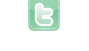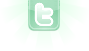## Gradually decrease and increasing speed

I am a Comp sci 30 student and I am looking to finish my little project on increasing the Cloud's speed to go up gradually over time.
```public class Cloud extends Actor
{
private int speed = 4; //speed of the cloud
private int rightTurn = 525; //right side change direction
private int leftTurn = 240; //left side change direction

/**
* Move in the direction we are currently moving in. Turn if we reach a turning point.
*/
public void act()
{

setLocation (getX() + speed, getY());
Actor actor = getOneIntersectingObject(null); //check whether we are not intersecting with an actor
if (actor != null)
{
actor.setLocation ( actor.getX() + speed, actor.getY() ); //pengu will move with the cloud
}

if (atTurningPoint())
{
speed = -speed; //changes the direction of the cloud
}

}

public boolean atTurningPoint()
{
if (getX()<= leftTurn || getX()>= 525)
{
return true;
}
else
{
return false;
}
}

} ```
would you like the cloud's speed to increase indefinitely or cap out at a particular value?
lightbringer wrote...
I am a Comp sci 30 student and I am looking to finish my little project on increasing the Cloud's speed to go up gradually over time. << Code Omitted >>
For a smooth gradual increase of speed, you will need to track the location coordinates of the actor more precisely than by pixel coordinates (which greenfoot uses). That means either by decimal values (float or double) or by some factor of a pixel (int being divided by some factor). Greenfoot supplies a SmoothMover class to do just that. I also wrote my own smooth moving class called QActor (quality actor) which can be found in my Asteroids w/improved QActor superclass scenario. However, the following should suffice for your purposes:
```private static final int LEFT_LIMIT = 240, RIGHT_LIMIT = 525;
private static final int FACTOR= 250; // adjust as needed (higher values slow down the increase of speed)
private int speed = 4 * FACTOR;
private int x; // x-coordinate of actor multiplied by the factor
private int dir = 1; // direction: values to be only 1 or -1 (right or left)

{
x = getX() * FACTOR;
}

public void act()
{
speed++; // increase speed by a fraction
int lastX = getX();
Actor actor = getOneIntersectingObject(null);
x += dir*speed;
setLocation(x/FACTOR, getY());
if (actor != null) actor.setLocation(actor.getX()+getX()-lastX, actor.getY());
if ((dir == 1 && getX() >= RIGHT_LIMIT) || (dir ==-1 && getX() <= LEFT_LIMIT)) dir = -dir;
}```
cap out in a particular value
lightbringer wrote...
cap out in a particular value
```private static final int CAP = 8; // adjust as needed (standard speed)

// to replace line 14
if (speed / FACTOR < CAP) speed++;```
or
```private static final int CAP = 8 * FACTOR; // after FACTOR is declared; adjust as needed (factored speed)

// to replace line 14
if (speed < CAP) speed++;```